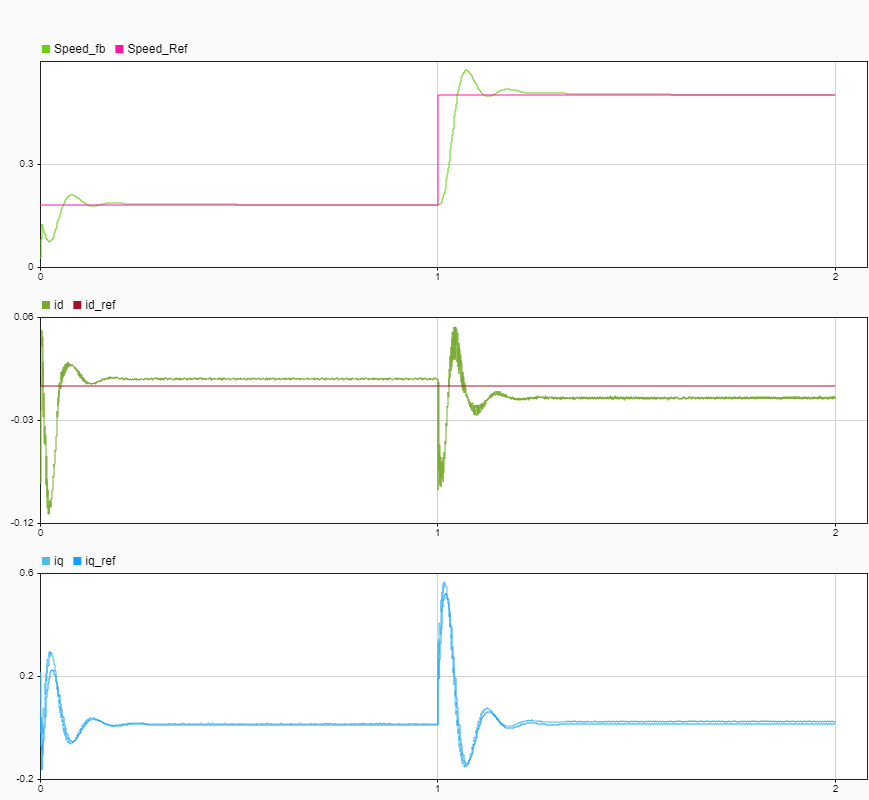# Generate Policy Block for Deployment

This example shows how to generate a Policy block ready for deployment from an agent object. You generate the policy from the agent in Train TD3 Agent for PMSM Control, then simulate it to validate its performance. The policy is simulated to validate performance. If Embedded Coder® is installed, a software-in-the-loop (SIL) simulation is run to validate the generated code of the policy.

In general, the workflow for the deployment of a reinforcement learning policy via a Simulink® model is:

1. Train the agent (see Train TD3 Agent for PMSM Control).

2. Generate a Policy block from the trained agent.

3. Replace the RL Agent block with the Policy block.

4. Configure the model for code generation.

5. Simulate the policy and verify policy performance.

6. Generate code for the policy, simulate the generated code, and verify policy performance.

7. Deploy to hardware for testing.

In this example, you do steps 2 through 6.

Load the motor parameters along with the trained TD3 agent.

`sim_data;`
```### The Lq is observed to be lower than Ld. ### ### Using the lower of these two for the Ld (internal variable) ### ### and higher of these two for the Lq (internal variable) for computations. ### ### The Lq is observed to be lower than Ld. ### ### Using the lower of these two for the Ld (internal variable) ### ### and higher of these two for the Lq (internal variable) for computations. ### model: 'Maxon-645106' sn: '2295588' p: 7 Rs: 0.2930 Ld: 8.7678e-05 Lq: 7.7724e-05 Ke: 5.7835 J: 8.3500e-05 B: 7.0095e-05 I_rated: 7.2600 QEPSlits: 4096 N_base: 3476 N_max: 4300 FluxPM: 0.0046 T_rated: 0.3471 PositionOffset: 0.1650 model: 'BoostXL-DRV8305' sn: 'INV_XXXX' V_dc: 24 I_trip: 10 Rds_on: 0.0020 Rshunt: 0.0070 CtSensAOffset: 2295 CtSensBOffset: 2286 CtSensCOffset: 2295 ADCGain: 1 EnableLogic: 1 invertingAmp: 1 ISenseVref: 3.3000 ISenseVoltPerAmp: 0.0700 ISenseMax: 21.4286 R_board: 0.0043 CtSensOffsetMax: 2500 CtSensOffsetMin: 1500 model: 'LAUNCHXL-F28379D' sn: '123456' CPU_frequency: 200000000 PWM_frequency: 5000 PWM_Counter_Period: 20000 ADC_Vref: 3 ADC_MaxCount: 4095 SCI_baud_rate: 12000000 V_base: 13.8564 I_base: 21.4286 N_base: 3476 T_base: 1.0249 P_base: 445.3845 ```
`load("rlPMSMAgent.mat","agent");`

### Generate Policy Block

Open the Simulink model used for training the TD3 agent.

```mdl_rl = "mcb_pmsm_foc_sim_RL"; open_system(mdl_rl);```

Open the subsystem containing the RL Agent block.

```agentblk = mdl_rl + ... "/Current Control/Control_System" + ... "/Closed Loop Control/Reinforcement Learning/RL Agent"; open_system(get_param(agentblk,"Parent"));```

To create a deployable model, you replace the RL Agent block with a Policy block.

Set the agent's `UseExplorationPolicy` property to false so the generated policy takes the greedy action at each time step. Generate the policy block using `generatePolicyBlock` and specify the name of the MAT-file containing the policy data for the block.

```% To ensure that the generated policy is greedy, % set UseExplorationPolicy to false agent.UseExplorationPolicy = false; % Specify the MAT-file name for the policy data fname = "PMSMPolicyBlockData.mat"; % Delete the file if it already exists if isfile(fname) delete(fname); end % Generate the block and the policy data generatePolicyBlock(agent,MATFileName=fname)```

Alternatively, you can generate the policy block can be generated by clicking Generate greedy policy block from the block mask. Use `open_system(agentblk)` to open the RL Agent block mask, or simply double-click the block.### Simulate the Policy

For this example, the Policy block has already replaced the RL Agent block inside of the `pmsm_current_control` model. This model has been configured for code generation, and the Policy block loads the trained policy from `PMSMPolicyBlockData.mat`.

```mdl_current_ctrl = "pmsm_current_control"; open_system(mdl_current_ctrl);```

Obtain the path of the policy block in the Simulink model.

```policyblk = mdl_current_ctrl + ... "/Current Control/Control_System" + ... "/Closed Loop Control/Reinforcement Learning/Policy";```

Use `open_system(policyblk)` to open the Policy block mask, or simply double-click the block.The model mcb_pmsm_foc_sim_policy references pmsm_current_control using a model reference block. Simulate the top-level model and plot the responses for the inner and outer control loops.

Setup the Simulation Data Inspector (SDI).

```Simulink.sdi.clear; Simulink.sdi.setSubPlotLayout(3,1);```

Open the model and get the path to the `Current Control` block.

```mdl_policy = "mcb_pmsm_foc_sim_policy"; open_system(mdl_policy); current_ctrl_blk = mdl_policy + "/Current Control";```

To temporarily change the model and easily run multiple simulations with such changes, `Simulink.SimulationInput` (Simulink) object.

`in = Simulink.SimulationInput(mdl_policy);`

Simulate the model with the current controller, run the simulation in normal mode

```in = setBlockParameter(in,current_ctrl_blk, ... "SimulationMode","Normal"); out_sim = sim(in);```

Get results from the latest SDI run.

`runSim = Simulink.sdi.Run.getLatest;`

Extract the outer control loop signals.

```speedSim = getSignalsByName(runSim,"Speed_fb" ); speedRefSim = getSignalsByName(runSim,"Speed_Ref");```

Plot both signals.

```plotOnSubPlot(speedSim ,1,1,true); plotOnSubPlot(speedRefSim,1,1,true);```

Extract the inner control loop signals.

```idSim = getSignalsByName(runSim,"id" ); iqSim = getSignalsByName(runSim,"iq" ); idRefSim = getSignalsByName(runSim,"id_ref"); iqRefSim = getSignalsByName(runSim,"iq_ref");```

Plot the extracted signals.

```plotOnSubPlot(idSim ,2,1,true); plotOnSubPlot(idRefSim,2,1,true); plotOnSubPlot(iqSim ,3,1,true); plotOnSubPlot(iqRefSim,3,1,true);```

Open the Simulation Data Inspector.

`Simulink.sdi.view;`### Validate Generated Code for Policy

If Embedded Coder is installed, the current controller model reference can be run in SIL mode. Running the current controller in SIL mode generates code for the current controller model, including the policy block.

The Simulink model is configured only on Windows, so display a message and stop execution when attempting to run on a different operating system.

```if ~ispc disp("The model ""pmsm_current_control"" is configured " + ... "for SIL simulations only on Windows system.") return end```

Simulate the model with the current controller.

Enable SIL mode.

```in = setBlockParameter(in,current_ctrl_blk, ... "SimulationMode","Software-in-the-loop");```

Simulate the model. Use `evalc` to capture the text output from code generation, for possible later inspection.

`txt_out = evalc("out_sil = sim(in)");`

Get results from the latest SDI run.

`runSIL = Simulink.sdi.Run.getLatest;`

Extract the speed response when run in SIL mode.

`speedSIL = getSignalsByName(runSIL,"Speed_fb");`

Compare the SIL response to the simulated response. The SIL response should be close to the response simulated in normal mode.

```speedSim.AbsTol = 1e-3; cr = Simulink.sdi.compareSignals(speedSim.ID,speedSIL.ID);```

Display the largest signal difference.

`cr.MaxDifference`
```ans = 5.0485e-05 ```

Open the Simulation Data Inspector.

`Simulink.sdi.view;`Once you are satisfied with the performance of the policy in simulation, you can use an appropriate target/hardware support package to deploy the policy to hardware.